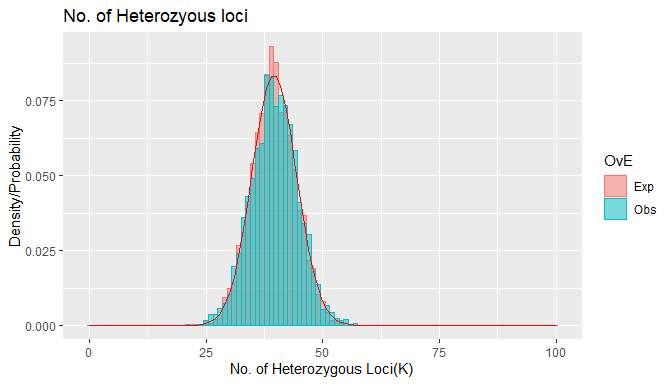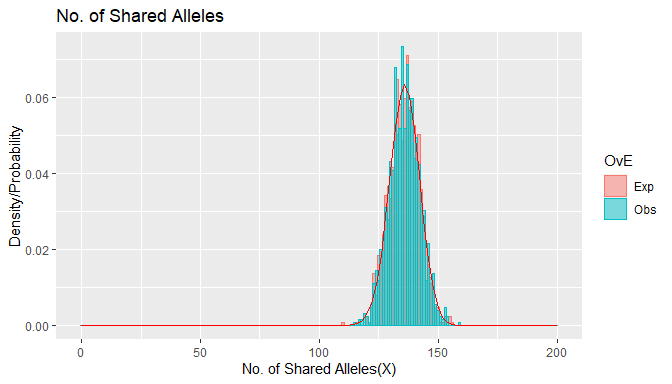Distribution Building and Comparison

This vignette will introduce you to how the package mixIndependR will build and compare the observed and expected distributions for number of heterozygous loci (K) and number of shared alleles (X).

With required parameters obtained from “basic genetics” part, observed and expected distributions can be built:

FreqHetero and DistHetero build the observed and expected distribution for number of heterozygous loci (K).

ObsDist_K<-FreqHetero(h)
ExpDist_K<- DistHetero(H)

FreqAlleleShare and DistAlleleShare build the observed and expected distribution for number of shared alleles (X).

ObsDist_X<-FreqAlleleShare(AS)
ExpDist_X<-DistAlleleShare(e)

ComposPares convert the above distributions into a format suitable for visualization. With the trans=TRUE, the observed frequencies and the expected density would be in separate columns. If trans=FALSE, two variables would be “OvE” (denoting the status of “observed or expected”) and “frequencies”.

df_K <- ComposPare_K(h,ExpDist_K,trans = F)
df_X <- ComposPare_X(AS,ExpDist_X,trans = F)